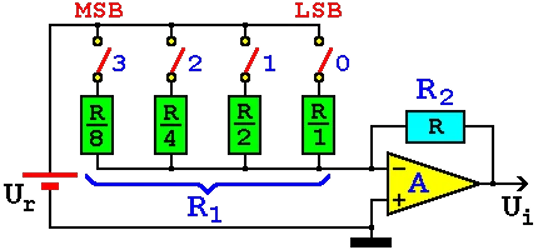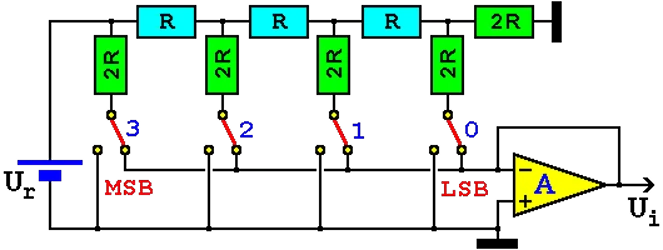# 7.1.2. D/A ConverterDespite the many benefits of becoming a digitally coded signals, however, is an analog display in many cases better, as for example when examining the overall scale of the measuring instrument can quickly determine the position of the hands, where the instrument is about the size measured in relation to the observed measurement range. In this way we can quickly look at the overall state of a system. Of course, the accuracy of such readings is questionable especially if small changes are important.

Unlike the previous examples above, the regulatory technique for analog circuit executive nature need to convert digital signals to analog. For example, D/A converter to provide the voltage at the output of the control unit will indicate the power of an electric motor in order to control the electric motor controls. Generally, the control mechanism that allows the output voltage from the D/A converter monitors and / or manages the mechanical displacement is called an ACTUATOR. Thus, the main task of digital-to-analog (D/A) converter is the management and monitoring is done in the control device or callback data by the A/D converter. From the above it can be concluded that the A/D and D/A converters basic premise of REGULATION. Methods of realization of transducers are very diverse but generally is used by network or networks of resistance current sources and so-called parallel conversion when the one-step binary combination given output signal.Figure 7.1.5 Value of the resistor in the D/A converter.

To parallel the name derives from the fact that at the same time to bring all the switches corresponding bits from LSB to MSB bits (bits maximum and minimum weight values).

Switches manage binary combinations, in the example with the image size of 4 bits. Switches can be performed by electromechanical or electronic, more often the latter.

The ratio of output and input (reference) voltage can be displayed simplified mathematical expression:Property operative amplifier with very high input resistance and very low output resistance allows the above simple mathematical analysis. The amplifier does not work in the classic sense that amplifies the input signal, but to changes in the relations of resistance at the output of a zoom invariant reference voltage (input) in relation to the number of resistor whose value decreases by a factor of 2n in the denominator.

The total value of the parallel resistor circuit is R1 and varies in accordance with the position of switches that are managed by the binary combinations. R2 value does not change.

Gain of operative amplifier depends solely on the relation resistance of R2 and R1, and the negative sign indicates that changes will be made phase output voltage relative to the input. Resistance R1 represents the parallel combination of resistance, which include switches 0-3 in the circuit as a function of the binary combinations that govern them.

Size of reference voltage Ur must be such that the output voltage does not exceed Ui supply voltage of operative amplifier. The following table shows how to change the amplification circuit according to changes in the resistance R1 and the binary combinations that control switches.

 Number R1 A 0000 - 0 0001 R -1 0010 R/2 -2 0100 R/4 -4 1000 R/8 -8 0011 R/3 -3 1100 R/12 -12 1111 R/15 -15

If you would like to implement 12-bit converter in the R1 branch should be located 12 resistors with switches that would value decreased by a factor of 2n sequentially from LSB to MSB.

Lack circuit is shown in the fact that they require exact relation between individual resistors. Therefore, rather resort to the so-called R-2R resilient network that has only two values of resistors that is technologically simpler to produce.Figure 7.1.6 D/A converter with R-2R of resistor network.

The circuit in Figure no gain compared to the previous one, but his operative amplifier circuit according to figure out just forward of resistant network without amplification and with properties of high input and low output resistance completely separating resistive network of circuits that follow in its output, and a full circuit makes it very stable. Besides the version displayed of resistor network used several similar.

Modern CMOS technology allows the production of D/A converters with a weight where capacitors used capacitor voltage dividers. This technology is quite widespread due to high accuracy, but it has the disadvantage of not using parallel operation.Citing of this page: Radic, Drago. " IT - Informatics Alphabet " Split-Croatia. {Date of access}. . Copyright © by Drago Radic. All rights reserved. | Disclaimer